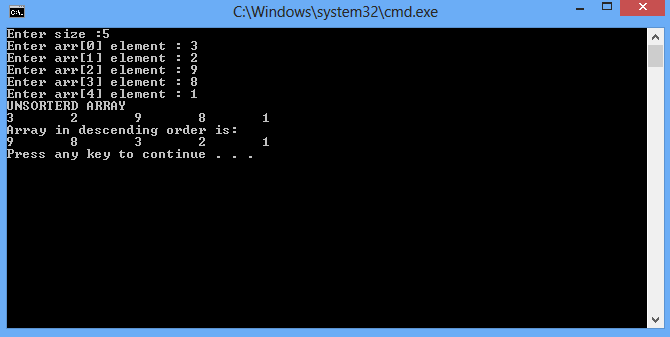# Introduction to Arrays

In this part you will learn: 1. Arrays 2. Sorting using arrays 3. Constants 4. Showing output In this tutorial I will teach you how to use arrays. What is an Array ? An array is a set of variables which you can declare in a single statement. For example if you want to make a result calculating program you need to declare the total number of students first. So instead of declaring student1, student2 etc you can write int students; in this way we declared 40 variables in a single statement. Sorting the array in descending order Basic Step: Open Dev C++ then File > new > source file and start writing the code below.
`#include<stdio.h>#include<conio.h>#define SIZE 1000`
These are the header files that we need for coding. The main header is stdio.h which provides us with most of the functions for coding in C and the second header files is just for using a function which can pause the screen until the user hits any key. the #define statement is used to declare a constant variable.
`int main(){	int input,i,x,y,j,temp = 0,index;	int arr[SIZE]={0};`
Now writing the main function. We will declare variables for taking input, counters for loops and index variable to track the index of the array. Then finally we will declare and array using the constant SIZE variable that has a value of 1000.
`	printf("Enter size :");	scanf("%d",&input);`
Now taking input of size to limit the array size. ( REMEMBER we can’t set the size of array in run time so we have to declare the constant SIZE first and then limit it by user input.)
`for (i = 0;i < input; i++)	{		printf("Enter arr[%d] element : ",i);		scanf("%d",&arr[i]);	}`
Running a for loop to take the input in array variable. We will enter the element one by one on every iteration of the loop.
`	printf("UNSORTERD ARRAY\n");	for (i = 0;i < input;i++)	{		printf("%d\t",arr[i]);	}}`
After taking the input from the user we will first print to see the elements. To print the elements we will start the loop from 0 to the point of limit which is given by the user. We simple print the array with the index of I which is the loop counter starting form 0. The loop is starting from 0 because in an array the elements are stored from 0 to one less than the limit for example int arr; there will be 3 elements in the array and we can access it by arr; arr; arr;
`for(x = 0 ;x<input;x++){	for(y=x;y<input;y++){			if(arr[x]<arr[y]){				temp =arr[x];				arr[x]=arr[y];				arr[y]=temp;			}		}//end inner for	}//end outer for`
Now to sort the numbers we will run two loops. We will compare each number with all the numbers in the array and then adjust it’s position accordingly in descending order. So first of all making 2 nested loops outer one running form 0 to ONE LESS THAN INDEX and inner one running from outer loop counter value to ONE LESS THAN INDEX. After that we simply write an if condition that if the 1st value is less than the second value then store it in temporary variable and swap it with the greater number. After that store the temporary variable back to the variable which is picked up. So what we do is we simply compare each number in array with the complete array and if the number is less we simply swap.
`printf("\nArray in descending order is:\n");	for(j=0;j<input;j++){		printf("%d\t",arr[j]);	}	printf("\n");	return 0;}`
In the end we will run a loop to print our already sorted array and then we end our program. Execute > compile then Execute > run Output